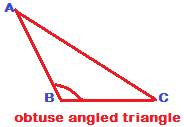## Triangle Inequality Property of sides

Very important:

The triangle inequality property

According to the triangle inequality property

In any triangle, the length of a side should be less than the sum and greater than the difference of the lengths of the other two sides

Simply put, the length of a side should lie between the difference and the sum of the other two sides.The triangle inequality property of sides can be written for all three sides as follows

b – c < a < b + c

c – a < b < c + a

a – b < c < a + b

Example:

Can a triangle be formed by sides whose lengths are 3, 4 and 5?

Answer:

Check the triangle inequality property of sides on ‘5’as follows

Is 4 – 3 < 5 < 4 + 3,

Is 1 < 5< 7?

Yes, it is.

Therefore triangle inequality property confirms formation of a triangle if the lengths of the sides are 3, 4 and 5.

Note:

It is sufficient to check whether the triangle inequality property holds on largest length, here 5. If it does, then on other lengths, it will certainly do; needs no verification.

Example 2:

Can a triangle be formed if the sides are of lengths 5, 10 and 15?

Solution:

Check triangle inequality property on 15, the largest number

Is 10 – 5 < 15 < 10 + 5

Is 5 < 15 < 15?

Though

5 < 15 is true, but 15 < 15 is false.

Since triangle inequality property does not hold, therefore a triangle cannot be formed if the sides have lengths 5, 10 and 15.

The length of the third side cannot be as much as 15, if the other two sides are 5 and 10.

Example 3:

Two sides in an isosceles triangle are 3 and 7. What is the length of the third side?

Solution:

Since the triangle is isosceles, therefore any two sides must be equal in length.

Therefore the third side could be either 3 or 7

Therefore, for the triangle to be isosceles:

the side-triplets must be 3, 3, 7 or 3, 7, 7

Apply triangle inequality property to find out which of the two 3 or 7 is indeed the length of the third side

With the triplet 3, 3 and 7, it is

3 – 3 < 7 < 3 + 3,

0 < 7 < 6, which is not correct.

With the other triplet 3, 7, 7, the triangle inequality property is

7 – 3 < 7 < 7 + 3, i.e.

4 < 7 < 10, which is right.

Therefore, for the triangle to form, the third side has to be of length 7

Short-cut:

Take third side as c

Now, 7 – 3 < c < 7 + 3

4 < c < 10

Between 3 and 7, it is 7 that lies between 4 and 10.

Therefore, third side should be 7.

Triangle Inequality property of sides in an acute triangleIn words, the triangle inequality property on sides in an acute angled triangle is

The square of any side is less than the sum of the squares of the other two sides in an acute triangle.

Mathematically written, the property in an acute triangle is

AB2 + BC2 > AC2

AC2 + BC2 > AB2

AB2 + AC2 > BC2

Example:

If the lengths of the sides in a triangle are 4, 5 and 6, then what type of triangle is formed?

Solution:

Let us check the triangle inequality property

Is 42 + 52 > 62 ?

Is 16 + 25 > 36?

Is 41 > 36,

Yes, it is.

Therefore, sides whose lengths are 4, 5 and 6 will form an acute triangle.

Every angle in the triangle is acute.

Triangle equality property in a right angled triangle

The sum square of the hypotenuse is equal to the sum of the squares of the other two sides

(Hypotenuse is the longest side opposite the right angle, while the other two sides containing the right angle are called legs of the right triangle)

The triangle equality property in a right triangle is the very famous Pythagoras Theorem. And it is

AB2 + BC2 = AC2Example

Find whether a right triangle is formed if the sides are of lengths 9, 40 and 41?

Solution:

Let us check the Pythagorean theorem as below

Is 92 + 402 = 412

Is 81 + 1600 = 1681

Is 1681 = 1681

Yes, it is.

This proves the sides 9, 40 and 41 form a right triangle.

The right angle is formed between the sides 9 and 40, or opposite the side 41.

Triangle Inequality property in an obtuse angled triangleThe Triangle inequality property in obtuse angled triangle is

The square of the longest side in an obtuse triangle is greater than the sum of the squares of the other two sides.

The inequality is

AB2 + BC2 > AC2

if AC is the longest side opposite the obtuse angle.

Example:

What type of triangle is formed if the lengths of its sides are 6, 8 and 11?

Solution:

Check the triangle inequality property as below

Is 112 > 62 +82

Is 121 > 100

Yes, it is.

Therefore, the triangle contains one obtuse angle.

It is formed opposite the side whose length is 11

Triangle inequality property worksheet